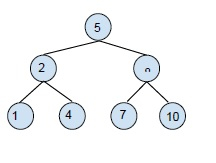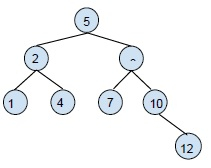# Binary Search Tree - Search and Insertion Operations in C++

Binary search tree (BST) is a special type of tree which follows the following rules −

• left child node’s value is always less than the parent Note
• right child node has a greater value than the parent node.
• all the nodes individually form a binary search tree.

Example of a binary search tree (BST) −A binary search tree is created in order to reduce the complexity of operations like search, find minimum and maximum.

## Search operation in BST

Performing a search in a binary search tree,

We need to search for a key in the tree. For this, We will compare the key with the root node of the tree.

If key equals to root node, the key is found.

If the value of the key is greater than the root node, take the right subtree and search for the key.

If the value of the key is less than the root node, take left subtree and search for the key.

### Example

Live Demo

#include<stdio.h>
#include<stdlib.h>
struct node{
int key;
struct node *left, *right;
};
struct node *newNode(int item){
struct node *temp = (struct node *)malloc(sizeof(struct node));
temp->key = item;
temp->left = temp->right = NULL;
return temp;
}
void traversetree(struct node *root){
if (root != NULL){
traversetree(root->left);
printf("%d \t", root->key);
traversetree(root->right);
}
}
struct node* search(struct node* root, int key){
if (root == NULL || root->key == key)
return root;
if (root->key < key)
return search(root->right, key);
return search(root->left, key);
}
struct node* insert(struct node* node, int key){
if (node == NULL) return newNode(key);
if (key < node->key)
node->left = insert(node->left, key);
else if (key > node->key)
node->right = insert(node->right, key);
return node;
}
int main(){
struct node *root = NULL;
root = insert(root, 23);
insert(root, 15);
insert(root, 12);
insert(root, 17);
insert(root, 32);
insert(root, 29);
insert(root, 45);
printf("The tree is :\n");
traversetree(root);
printf("\nSearching for 12 in this tree ");
if(search(root , 12))
printf("\nelement found");
else
return 0;
}

### Output

The tree is :
12 15 17 23 29 32 45
Searching for 12 in this tree
element found

## Insertion operation in BST

Insertion operation in a BST takes place at the leaf node of the tree for insertion we will start the comparison of the node with the root node and find the correct position of the node and then place it. The following example will make it more clear to you.Inserting 12 to this BST.

We will compare 12 with root node 12 > 5, it belongs to the right subtree.

Compare 12 with the right child node 12 > 8, it belongs to the right of the right sub child.

Compare 12 with the right sub child of right subtree 12 >10, its position is the right of this node.

The new tree formed will be,## Example

Live Demo

#include<stdio.h>
#include<stdlib.h>
struct node{
int key;
struct node *left, *right;
};
struct node *newNode(int item){
struct node *temp = (struct node *)malloc(sizeof(struct node));
temp->key = item;
temp->left = temp->right = NULL;
return temp;
}
void traversetree(struct node *root){
if (root != NULL){
traversetree(root->left);
printf("%d \t", root->key);
traversetree(root->right);
}
}
struct node* insert(struct node* node, int key){
if (node == NULL) return newNode(key);
if (key < node->key)
node->left = insert(node->left, key);
else if (key > node->key)
node->right = insert(node->right, key);
return node;
}
int main(){
struct node *root = NULL;
root = insert(root, 23);
insert(root, 15);
insert(root, 12);
insert(root, 17);
insert(root, 32);
insert(root, 29);
printf("The tree is :\n");
traversetree(root);
printf("\nInseting 45 to the tree\n");
insert(root, 45);
printf("Tree after insertion is :\n");
traversetree(root);
return 0;
}

## Output

The tree is :
12 15 17 23 29 32
Inserting 45 to the tree
Tree after insertion is :
12 15 17 23 29 32 45